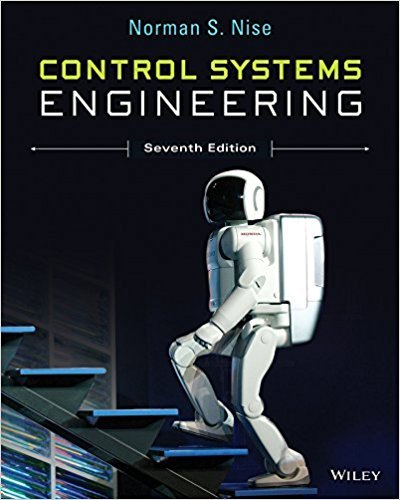×
Get Full Access to Control Systems Engineering - 7 Edition - Chapter 4 - Problem 66
Get Full Access to Control Systems Engineering - 7 Edition - Chapter 4 - Problem 66

×

# A dc-dc converter is a device that takes as an input anISBN: 9781118170519 162

## Solution for problem 66 Chapter 4

Control Systems Engineering | 7th Edition

• Textbook Solutions
• 2901 Step-by-step solutions solved by professors and subject experts
• Get 24/7 help from StudySoup virtual teaching assistantsControl Systems Engineering | 7th Edition

4 5 1 398 Reviews
20
5
Problem 66

A dc-dc converter is a device that takes as an input an unregulated dc voltage and provides a regulated dc voltage as its output. The output voltage may be lower (buck converter), higher (boost converter), or the same as the input voltage. Switching dc-dc converters have a semiconductor active switch (BJT or FET) that is closed periodically with a duty cycle d in a pulse width modulated (PWM) manner. For a boost converter, averaging techniques can be used to arrive at the following state equations (Van Dijk, 1995): L diL dt 1 duc Es C duC dt 1 diL uC R where L and C are, respectively, the values of internal inductance and capacitance; iL is the current through the internal inductor; R is the resistive load connected to the converter; Es is the dc input voltage; and the capacitor voltage, uC, is the converters output. a. Write the converters equations in the form x_ Ax Bu y Cx assuming d is a constant. b. Using the A, B, and C matrices of Part a, obtain the converters transfer function UCs Ess :

Step-by-Step Solution:
Step 1 of 3

FEBRUARY 16, 2016­ NO CLASS FEBRUARY 18, 2016 Conditional Expression  Shorthand for if statements  Variable=(condition) expWhenTrue : expWhenFalse  Basically if the condition is met then the first exp is printed, if not than the second is printed  Example: o If the grade is greater than 70 the program will print “Passed” o If the grade is less than 70 the program will print “Failed” Double/Float Comparison  Doubles and floats are not exact, for example: Expected (or entered) value Actual (how it is actually stored) 0.7 0.699999999999999999994512575 0.3 0.29999999999999999999974578 0.0

Step 2 of 3

Step 3 of 3

##### ISBN: 9781118170519

Control Systems Engineering was written by and is associated to the ISBN: 9781118170519. Since the solution to 66 from 4 chapter was answered, more than 308 students have viewed the full step-by-step answer. This textbook survival guide was created for the textbook: Control Systems Engineering, edition: 7. The answer to “A dc-dc converter is a device that takes as an input an unregulated dc voltage and provides a regulated dc voltage as its output. The output voltage may be lower (buck converter), higher (boost converter), or the same as the input voltage. Switching dc-dc converters have a semiconductor active switch (BJT or FET) that is closed periodically with a duty cycle d in a pulse width modulated (PWM) manner. For a boost converter, averaging techniques can be used to arrive at the following state equations (Van Dijk, 1995): L diL dt 1 duc Es C duC dt 1 diL uC R where L and C are, respectively, the values of internal inductance and capacitance; iL is the current through the internal inductor; R is the resistive load connected to the converter; Es is the dc input voltage; and the capacitor voltage, uC, is the converters output. a. Write the converters equations in the form x_ Ax Bu y Cx assuming d is a constant. b. Using the A, B, and C matrices of Part a, obtain the converters transfer function UCs Ess :” is broken down into a number of easy to follow steps, and 183 words. The full step-by-step solution to problem: 66 from chapter: 4 was answered by , our top Engineering and Tech solution expert on 11/23/17, 05:05AM. This full solution covers the following key subjects: voltage, converter, converters, output, Input. This expansive textbook survival guide covers 13 chapters, and 734 solutions.

Unlock Textbook Solution